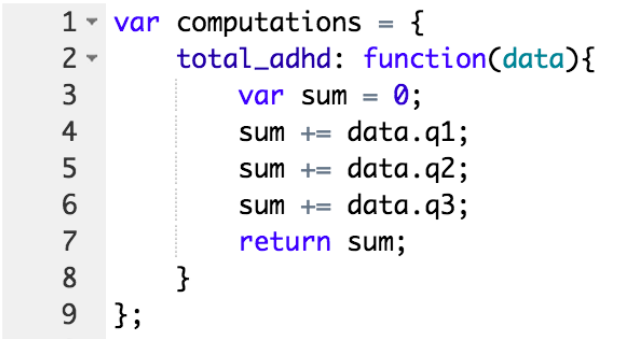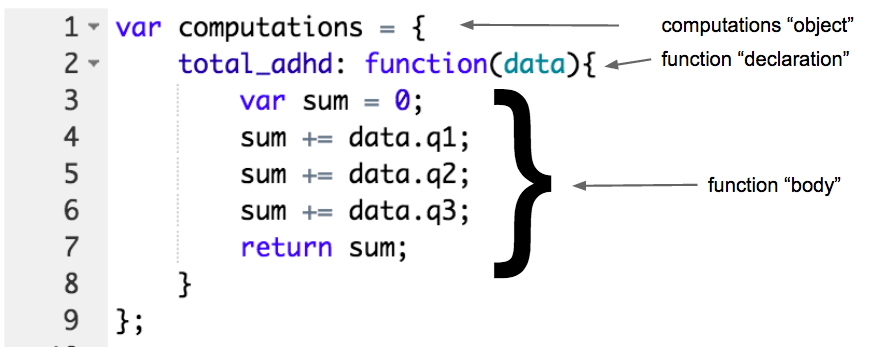A computation is a calculation based on answers of a client.

What can you do with a computation?

• Calculate the 'total score' on a questionnaire
• Give an advice that depends on the score a client gets on a questionnaire
• Give back the score on several domains to the client and therapist
• Make a piece of content within a session dependent on a calculated score
• Trigger a piece of content based on the outcome of a calculation or questionnaire

For example, you can calculate BMI (Body Mass Index), and give back an advice to the client based on whether they scored underweight, normal weight, or overweight.

What does a calculation look like?

A computation is a piece of code (javascript) in which you write a calculation based on the question(s) in your session.If you want, you can learn more about code. This will help you to get more creative with your computations. It is based on javascript, so most rules of javascript will apply. More on that later.

Understanding the parts of a computation

A computation consists of a couple of core parts. They are the following:The computations 'object' The total range of calculations, from the beginning bracket { to the end bracket } and the finishing semicolon, is called the 'object'. This is the code that the computer will run through. We will put all the computations in here. The function declaration A function is like a little machine in which we build the calculation we want the computer to run through. We give it a name, let the computer know that the function starts at the "name: function" and that we want it to use "(data)". Data The command 'data'  is used to refer to the answers saved in the document. This way, the data the client enters is used in the computation and the score of that particular client is given back. The function body The function body is the content of the machine, the actual calculation.